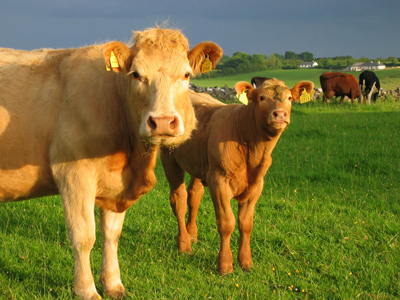Farmer Bull has 20 cows. How many sheep does he have?

# Ratio 2 (Medium)

Welcome to this, our second Medium level Eleven Plus maths quiz on ratios. As you will know if you have played the first, ratios are ways to express or show proportions of amounts. They are quite similar to fractions but slightly different.

The best way to explain is with an example:

Let’s say that the ratio of vegetarians to meat-eaters is 2:5. That means that for every 2 vegetarians there are 5 meat-eaters.

You can also represent this with fractions: In the above example 27 are vegetarians and 57 are meat-eaters. That’s because 2 + 5 = 7.

Okay then, now we’ve refreshed our memories, it’s time to get on with the quiz. Good luck!

1.
The ratio of cheese sandwiches to ham sandwiches is 7:5. If there are 36 sandwiches in all, how many ham sandwiches are there?
21
18
15
12
7 + 5 = 12 so 712 of the sandwiches are cheese and 512 are ham. 36 ÷ 12 = 3 so each unit in the ratio represents 3 sandwiches. 3 x 7 = 21 and 3 x 5 = 15. There are 21 cheese sandwiches and 15 ham sandwiches
2.
Farmer Bull has 20 cows. How many sheep does Farmer Bull have if the ratio of cows to sheep is 5:6?
22
24
26
28
5:6 = 1:1.2 (dividing by 5). This means that there is 1 cow for every 1.2 sheep ∴ there are 1.2 as many sheep as there are cows = 1.2 × 20 = 24. CHECK 20:24 = 1:1.2 (dividing by 20). Your answer MUST preserve (keep) the same ratio. ALWAYS try to REDUCE your ratios so as to get a '1' in one of the terms of the ratio. Incidentally, 20 + 24 = 44 animals in all!
3.
The ratio of red roses to white roses is 7:9. What fraction of the roses are white?
98
716
78
916
7 + 9 = 16. You DON'T have to know what the total number of items is in order to find the fractional amounts of each type of item
4.
Seventy photographs are to be displayed in a gallery. If the ratio of portrait pictures to landscape pictures has to be 3:4, how many portrait pictures and landscape pictures have to be selected?
30 portrait pictures and 40 landscape pictures
35 portrait pictures and 35 landscape pictures
25 portrait pictures and 45 landscape pictures
45 portrait pictures and 25 landscape pictures
3 + 4 = 7 ∴ the fractional parts are 37 × 70 = 30 portrait pictures and 47 × 70 = 40 landscape pictures. CHECK 30:40 = 3:4 (dividing by 10). Your answer MUST preserve (keep) the same ratio
5.
If the ratio of swimmers to non-swimmers is 9:2, which of the following statements is correct?
There are more swimmers than non-swimmers
There are an equal amount of swimmers and non-swimmers
There are more non-swimmers than swimmers
There is not enough information to answer the question
9 is greater than 2 and it represents the number of swimmers. There are clearly more swimmers than non-swimmers
6.
How else can the ratio 9:24 be written?
3:8
4:12
1:3
18:46
9:24 = 3:8 (dividing by 3)
7.
How else can the ratio 1.75:2 be written?
7:8
6:7
5:6
4:5
1.75:2 = 7:8 (multiplying by 4)
8.
Which of the following is not a way of expressing the ratio 5:6?
10:12
25:30
45:54
60:78
10:12 is the same as 5:6 (multiply by 2), 25:30 is the same as 5:6 (multiply by 5), 45:54 is the same as 5:6 (multiply by 9). 60:78 is NOT the same as 5:6 – the 5 has been multiplied by 12 but the 6 has been multiplied by 13
9.
The following ratios show the ratio of wins to defeats for four football teams. If all four teams have played the same number of games, which team has won the most often?
1:1
9:10
21:17
14:24
1:1 represents an equal number of wins and defeats. 9:10 and 14:24 both represent fewer wins than defeats. Only 21:17 represents more games won than lost
10.
47 of the children in a class are girls. What is the ratio of girls to boys in the class?
3:4
4:3
4:7
7:4
If 47 of the children in a class are girls, then 37 of the children must be boys
Author:  Frank Evans